# Fraction calculator

The calculator performs basic and advanced operations with fractions, expressions with fractions combined with integers, decimals, and mixed numbers. It also shows detailed step-by-step information about the fraction calculation procedure. Solve problems with two, three, or more fractions and numbers in one expression.

## Result:

### 2/3 * 5 = 10/3 = 3 1/3 ≅ 3.3333333

Spelled result in words is ten thirds (or three and one third).

### How do you solve fractions step by step?

1. Multiple: 2/3 * 5 = 2 · 5/3 · 1 = 10/3
Multiply both numerators and denominators. Result fraction keep to lowest possible denominator GCD(10, 3) = 1. In the following intermediate step, the fraction result cannot be further simplified by canceling.
In other words - two thirds multiplied by five = ten thirds.

#### Rules for expressions with fractions:

Fractions - simply use a forward slash between the numerator and denominator, i.e., for five-hundredths, enter 5/100. If you are using mixed numbers, be sure to leave a single space between the whole and fraction part.
The slash separates the numerator (number above a fraction line) and denominator (number below).

Mixed numerals (mixed fractions or mixed numbers) write as integer separated by one space and fraction i.e., 1 2/3 (having the same sign). An example of a negative mixed fraction: -5 1/2.
Because slash is both signs for fraction line and division, we recommended use colon (:) as the operator of division fractions i.e., 1/2 : 3.

Decimals (decimal numbers) enter with a decimal point . and they are automatically converted to fractions - i.e. 1.45.

The colon : and slash / is the symbol of division. Can be used to divide mixed numbers 1 2/3 : 4 3/8 or can be used for write complex fractions i.e. 1/2 : 1/3.
An asterisk * or × is the symbol for multiplication.
Plus + is addition, minus sign - is subtraction and ()[] is mathematical parentheses.
The exponentiation/power symbol is ^ - for example: (7/8-4/5)^2 = (7/8-4/5)2

#### Examples:

subtracting fractions: 2/3 - 1/2
multiplying fractions: 7/8 * 3/9
dividing Fractions: 1/2 : 3/4
exponentiation of fraction: 3/5^3
fractional exponents: 16 ^ 1/2
adding fractions and mixed numbers: 8/5 + 6 2/7
dividing integer and fraction: 5 ÷ 1/2
complex fractions: 5/8 : 2 2/3
decimal to fraction: 0.625
Fraction to Decimal: 1/4
Fraction to Percent: 1/8 %
comparing fractions: 1/4 2/3
multiplying a fraction by a whole number: 6 * 3/4
square root of a fraction: sqrt(1/16)
reducing or simplifying the fraction (simplification) - dividing the numerator and denominator of a fraction by the same non-zero number - equivalent fraction: 4/22
expression with brackets: 1/3 * (1/2 - 3 3/8)
compound fraction: 3/4 of 5/7
fractions multiple: 2/3 of 3/5
divide to find the quotient: 3/5 ÷ 2/3

The calculator follows well-known rules for order of operations. The most common mnemonics for remembering this order of operations are:
PEMDAS - Parentheses, Exponents, Multiplication, Division, Addition, Subtraction.
BEDMAS - Brackets, Exponents, Division, Multiplication, Addition, Subtraction
BODMAS - Brackets, Of or Order, Division, Multiplication, Addition, Subtraction.
GEMDAS - Grouping Symbols - brackets (){}, Exponents, Multiplication, Division, Addition, Subtraction.
Be careful, always do multiplication and division before addition and subtraction. Some operators (+ and -) and (* and /) has the same priority and then must evaluate from left to right.

## Fractions in word problems:

• The machineThe machine was in operation for 9 tenths (in a fraction) of eight hours of working time. How long has he been running?
• Anne eats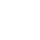Anne eats 3/4 cup of raisins each day. How many total cups of raisins does Annie eat in 14 days?
• Yellow tangs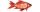In an aquarium 2/5 of the fish are surgeonfish.  Of these 3/4 are yellow tangs.  What fraction of all fish in the aquarium are yellow tangs?
• Berry SmoothieRory has 5/8 cup of milk. How much milk does she have left after she doubles the recipe of the smoothie? Berry Smoothie: 2 cups strawberries 1 cup blueberries 1/4 cup milk 1 tbsp (tablespoon) sugar 1/2 tsp (teaspoon) lemon juice 1/8 tsp (teaspoon) vanilla
• A recipeA recipe requires 3/4 cups of milk. Paula is making 1/2 of the recipe. How many cups will Paula use?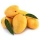A basket is full of 50 different kinds of fruits that has a ratio of 2:3:5. If the fruits are apple, mango, and guava, how many guavas are there?
• A supermarket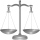A supermarket has 3 7/8 pounds of tangerines. Kelsey buys 1/2 of all the tangerines in the supermarket. How many pounds of tangerines did Kelsey buy?
• Hungry cat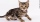John is a fully grown cat. He eats 2/3 of a can a day. How many cans of food does Tinker eat in a week? *
• Bathtub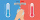Kevin has a bathtub shaped like a block. The bathtub measures 70 cm long, 45 cm wide and 50 cm high. Then the bathtub will be filled with clean water 3/5 of its height. The clean water needed is….
• Father's gardenJohn's father has a garden in the back yard. The dimensions of the garden are shown here. A green rectangle is shown. The width is labeled as 2 and two-thirds feet. The length is labeled as 7 and three-fourths feet. Calculate the area of the garden. You f
• Lengths of the poolMiguel swam 6 lengths of the pool. Mat swam 3 times as far as Miguel. Lionel swam 1/3 as far as Miguel. How many lengths did Mat swim?
• Ratio - proportion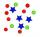Reduce the number 16 in proportion 3:2 5:4 11:8
• Toilets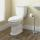Federal law requires that all residential toilets sold in the United States use no more than 1 3/5 gallons of water per flush. Before this legislation, conventional toilets used 3 2/5 gallons of water per flush. Find the amount of water saved in one year